## 2.3.4 Long-time behavior: Arrhenius-Néel law

Thermal fluctuations cause a random walk of the system in a multidimensional energy space, which ultimately produces a decay of the initial magnetization state. If the value of the thermal energy is comparable to the relevant energy barriers the system will be unstable and will randomly oscillate between the energy minima, presenting superparamagnetism. If this energy is small compared to the energy barrier value, the magnetization will stay in a local minimum during many precession periods and eventually will surmount the energy crest separating two energy minima and appear in the valley of another minimum. This phenomenon is known as thermal activation and is always present in any finite temperature experiment [Basso 00]. Due to the fact that the thermal switching over large energy barriers occurs rarely, the switching events are also known as infrequent events. Thermal activation can be also responsible for domain wall motion on pinning potentials due to defects [Chen 99]. The magnetization thermal stability poses an important problem and is a limitation from the point of view of magnetic-based technologies such as MRAM and magnetic recording. Due to this, the study of thermal activation is a subject of current interest. Moreover, the thermal activation is important in order to understand the magnetic relaxation and the dynamical coercivity phenomena from a fundamental point of view.

In the most general case, when temperature fluctuations are small as compared to energy barriers, the probability of magnetic switching is governed by the Arrhenius-Néel law: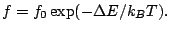(2.58)

The prefactor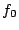is known as the attempt frequency and its inverse as switching timeconstant and has a value between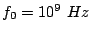and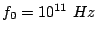for magnetic systems.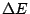is the energy barrier and its value is the energy of the saddle point minus the energy at the minimum. In a Stoner-Wohlfarth particle the energy barrier is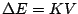, but in the general case, the calculation has to be done numerically in a multidimensional space (see Section 2.3.6). In experiments the attempt frequency is often taken as constant, but in general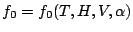.

To calculate the prefactor we need some simplifications. The Transition State Theory (TST) simplifies the thermodynamics of the system assuming that the trajectories pass through the saddle point. This theory has its origin in chemical reaction kinetics and was originally developed by H. Kramers [Kramers 40], who demonstrated that the prefactor can be calculated from the probability diffusion equation or Fokker-Planck equation (FPE). Due to this, the original energy minimum is known as reactive state and the final minimum as product state. The first evaluation in a magnetic system was for a single domain particles with uniaxial anisotropy and applied field parallel to the easy axis [Brown 63b]. In this case an asymptotic analytical expression was obtained from the smallest eigenvalue of the FPE equation: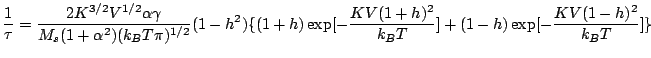(2.59)

Posteriorly, additional eigenvalues of the FPE equation were evaluated for a more accurate attempt frequency of a single domain particle [Coffey 95b] and applied field not parallel to the easy axis was included [Coffey 95a] .

For a general system and Intermediate to High Damping (IHD) case Langer developed an expression for the escape rate [Langer 68]:(2.60)

where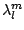are the eigenvalues of the energy Hessian (matrix of second derivatives of the energy function) at the initial minimum,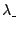is the negative eigenvalue of the Hessian at the saddle point,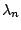are the positive eigenvalues of the Hessian at the saddle point,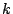is a dynamical term calculated linearizing the LLG equation and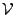is the volume of the saddle-point subspace. The volumegives the correct escape rate in the cases where symmetry exists (e.g. axial symmetry). Thus, the evaluation of the prefactor requires the calculation of the eigenvalues of the energy Hessian at the initial state and at the saddle point, and, again, except for simple systems it has to be calculated numerically. The general procedure to do this is to find the points where the energy gradient vanishes and then to find the nature of the stationary points. This calculation is affordable in small systems only and methods have been developed to overcome this problem [Chubykalo-Fesenko 05].

2008-04-04